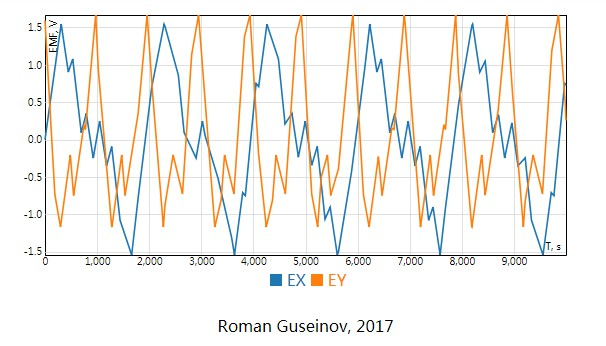/ Chart

# React Signals Plot component for geophysical data visualization## React Signals Plot

This is React Signals Plot component for geophysical data visualization.

The component supports 'on the fly' data compression. That's why you can use it for drawing line charts which contain millions of points. ReactSignalsPlot is an interactive component. You can use a mouse or touch to move and zoom.

## Install

`npm i --save react-signals-plot`

## Example

``````import React from 'react';
import ReactSignalsPlot from 'react-signals-plot';

const series = {
data: [
{
id: 'EX',
values: [
{ x: 1, y: 5 },
{ x: 2, y: 10 },
{ x: 3, y: 1 },
{ x: 4, y: 3 },
{ x: 5, y: 7 }
]
},
{
id: 'EY',
values: [
{ x: 1, y: 2 },
{ x: 2, y: 0 },
{ x: 3, y: 5 },
{ x: 4, y: 7 },
{ x: 5, y: 7 }
]
}
],
labels: {
x: 'X, seconds',
y: 'Y, volts'
}
};

class PlotExample extends React.Component {
render() {
return (
<ReactSignalsPlot
style={ { width: '100%', height: 400 } }
data={ series.data }
samplesLimit={ 300 }
labels={ series.labels }
interactive={ true }
/>
);
}
}
``````

## Scripts

1. `npm run build` - build component
2. `npm run dev` - start webpack-dev-server
3. `npm run test` - run unit tests

## GitHub#### React.js Examples

A nice collection of often useful examples done in React.js.

Latest
Tag